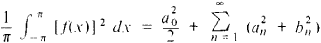# Parseval Equality

## Parseval Equality

an equation of the formwhere a0 an, and bn are the Fourier coefficients for f(x). It was established in 1805 by the French mathematician M. Parseval by assuming that trigonometric series could be termwise integrated. In 1896, A. M. Liapunov showed that the equality is valid if the function is bounded on the interval (—π, π) and if the integral ∫πf(x) dx exists. It was later proved that the Parseval equality holds for any function whose square is integrable. V. A. Steklov demonstrated that the Parseval equality is valid for series in other orthogonal systems of functions.

References in periodicals archive ?
By the Parseval equality we have [MATHEMATICAL EXPRESSION NOT REPRODUCIBLE IN ASCII].
d] ([psi], [chi]) can be evaluated either by direct integration or using convolution formula and Parseval equality for harmonic analysis on [S.
Since the spectrum is simple and discrete, then Parseval equality (6) yields for any sequence of values F([[lambda].
1] - f are zero and applying the Parseval equality (3.
and that f (b) = 0 in case b is left-scattered, we have the Parseval equality (see )
We will show that the Parseval equality for problem (1.
1 and the Helly theorems it is possible to get a spectral function and the Parseval equality for problem (1.
To get the Parseval equality, first assume that the function f satisfies the conditions:
Applying to this function the Parseval equality (3.
that is, the Parseval equality for any function f satisfying the above conditions 1.

Site: Follow: Share:
Open / Close